NCERT Solutions: Exponents & Powers - 2

# NCERT Solutions: Exponents & Powers - 2 - Notes | Study Mathematics (Maths) Class 8 - Class 8

 1 Crore+ students have signed up on EduRev. Have you?

USE OF EXPONENTS TO EXPRESS
Small Numbers in Standard Form
We know that” a number is said to be in the standard form, if it is expressed as the product of a number between 1 and 10 and integral power of 10.’’
So far, we have learnt to express large numbers in standard form. For example,
180,000,000,000 = 1.8 * 1011 [Decimal point is moved to left for 11 places]
We can also express very small numbers in standard form by moving the decimal point to the right.

Example: Express 0.000009 in standard form.
Solution: 0.000009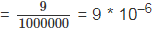[Decimal point is moved to right for 6 places]
Note: Writing a number in standard form means expressing it in scientific notation.

TRY THESE
Question 1. Write the following numbers in standard form.
(i) 0.000000564
(ii) 0.0000021
(iii) 15240000

Solution: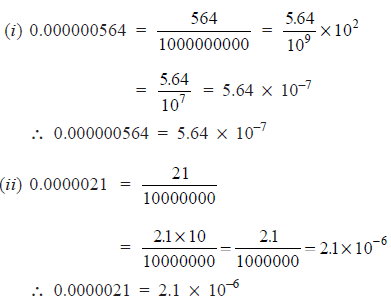(iii) 15240000 = 1524 * 10000
= 1.524 * 1000 * 10000
= 1.524 * 103 * 104 = 1.524 * 107
∴    15240000 = 1.524 * 107

Question 2. Write all the facts given in the standard form.
Solution: A number is said to be in the standard form when it is written as k * 10n, where
1 < k < 10
and ‘n’ is an integer.
A number expressed as the product of a number between 1 and 10 and an integral power of 10.

Example: Compare the size of a red blood cell which is 0.000007 m to that of a plant cell which is 0.0000129 m.
Solution: Size of red blood cell = 0.000007 m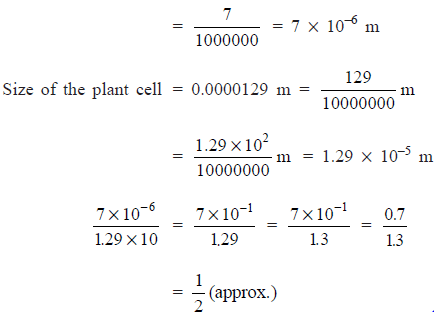Thus, the size of a red blood cell is half of the plant cell size.

EXERCISE 12.2
Question 1. Express the following numbers in standard form.
(i) 0.0000000000085
(ii) 0.00000000000942
(iii) 6020000000000000
(iv) 0.00000000837
(v) 31860000000
Solution: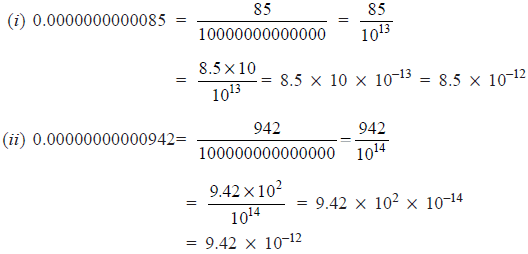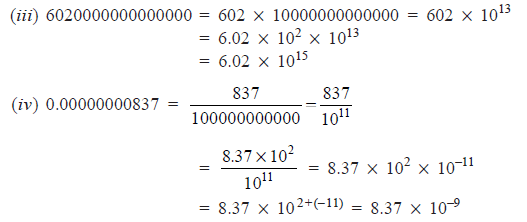(v) 31860000000 = 3186 * 10000000 = 3186 * 107
= 3.186 * 103 * 107
= 3.186 * 103+7 = 3.186 * 1010

Question 2. Express the following numbers in usual form.
(i) 3.02 * 10–6
(ii) 4.5 * 10
(iii) 3 * 10–8
(iv) 1.0001 * 10
(v) 5.8 * 1012
(vi) 3.61492 * 106

Solution:
(i)       3.02 * 10–6 = 302 * 10–2 * 10–6
= 302 * 10–8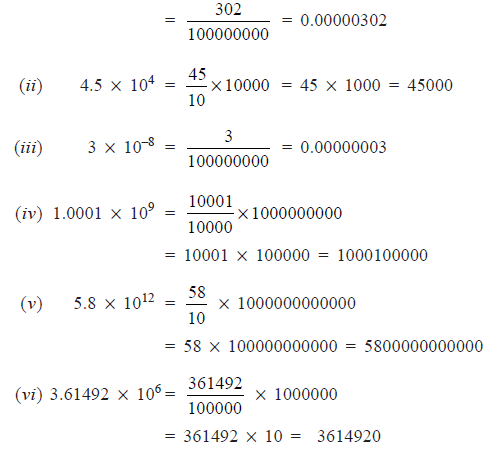Question 3. Express the number appearing in the following statements in standard form.
(i) 1 micron is equal to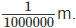(ii) Charge of an electron is 0.000,000,000,000,000,000,16 coulomb.
(iii) Size of a bacteria is 0.0000005 m.
(iv) Size of a plant cell is 0.00001275 m.
(v) Thickness of a thick paper is 0.07 mm.
Solution: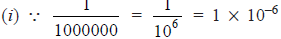∵ 1 micron = 1 * 10–6

(ii) ∵ 0.000,000,000,000,000,000,16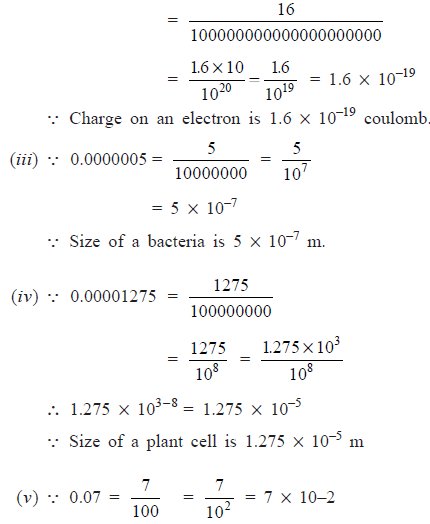∴  Thickness of a thick paper is 7 * 10–2 mm.

Question 4. In a stack there are 5 books each of thickness 20 mm and 5 paper sheets each of thickness 0.016 mm. What is the total thickness of the stack?
Solution:
∵ Thickness of one book = 20 mm
∴  Thickness of 5 books  = 5 * 20 mm = 100 mm
Again,
Thickness of 1 paper sheet = 0.016 mm
∴  Thickness of 5 paper sheets = 5 * 0.016 mm
= 0.080 m
∴                       Total thickness = 100 mm + 0.080 mm
= 100.08 mm = 1.0008 * 102 mm

The document NCERT Solutions: Exponents & Powers - 2 - Notes | Study Mathematics (Maths) Class 8 - Class 8 is a part of the Class 8 Course Mathematics (Maths) Class 8.
All you need of Class 8 at this link: Class 8

## Mathematics (Maths) Class 8

193 videos|360 docs|48 tests
 Use Code STAYHOME200 and get INR 200 additional OFF

## Mathematics (Maths) Class 8

193 videos|360 docs|48 tests

Track your progress, build streaks, highlight & save important lessons and more!

,

,

,

,

,

,

,

,

,

,

,

,

,

,

,

,

,

,

,

,

,

;